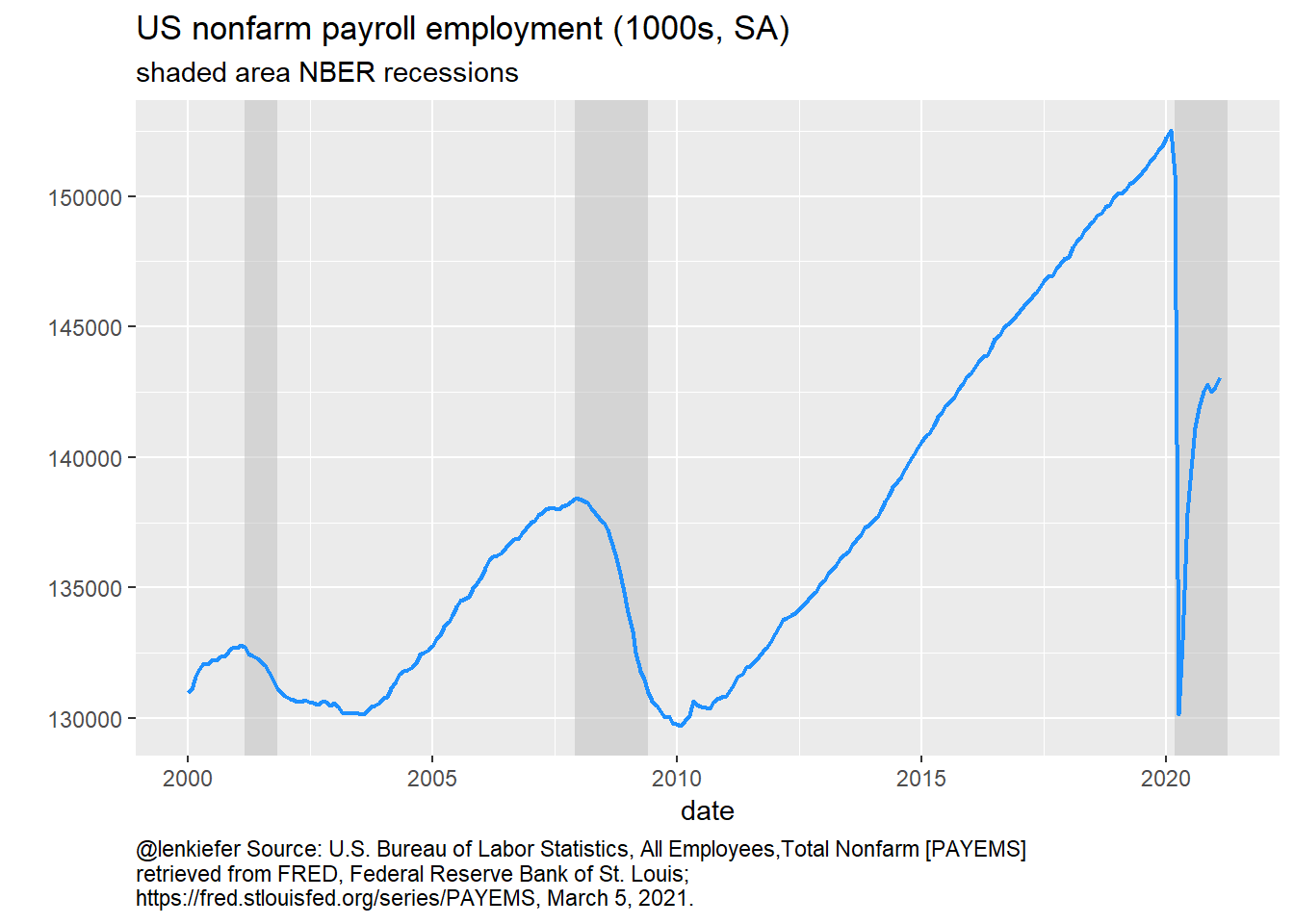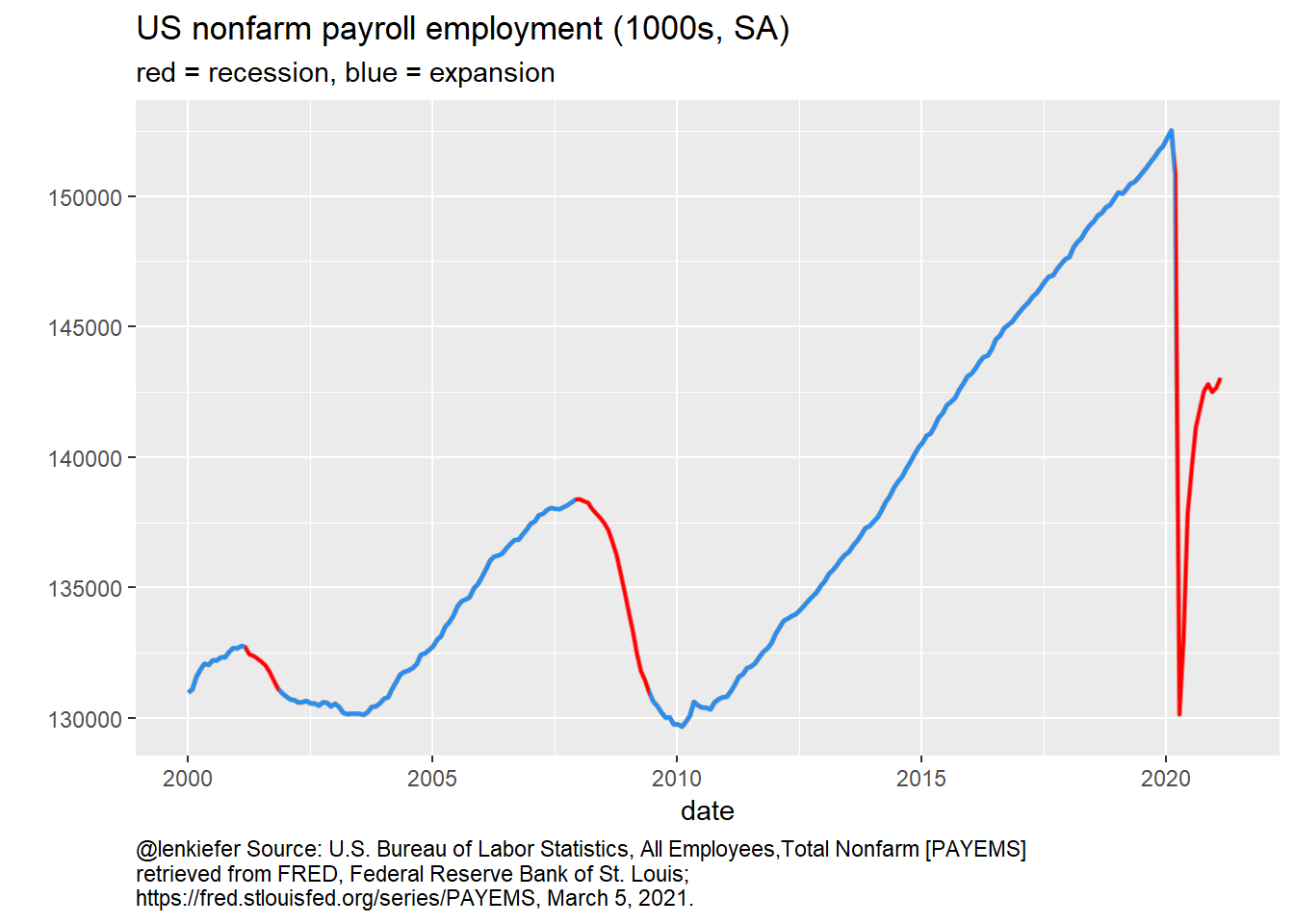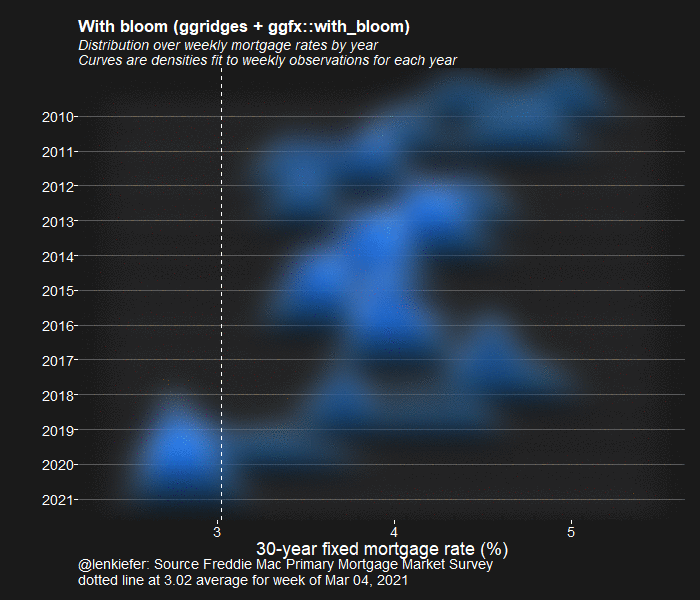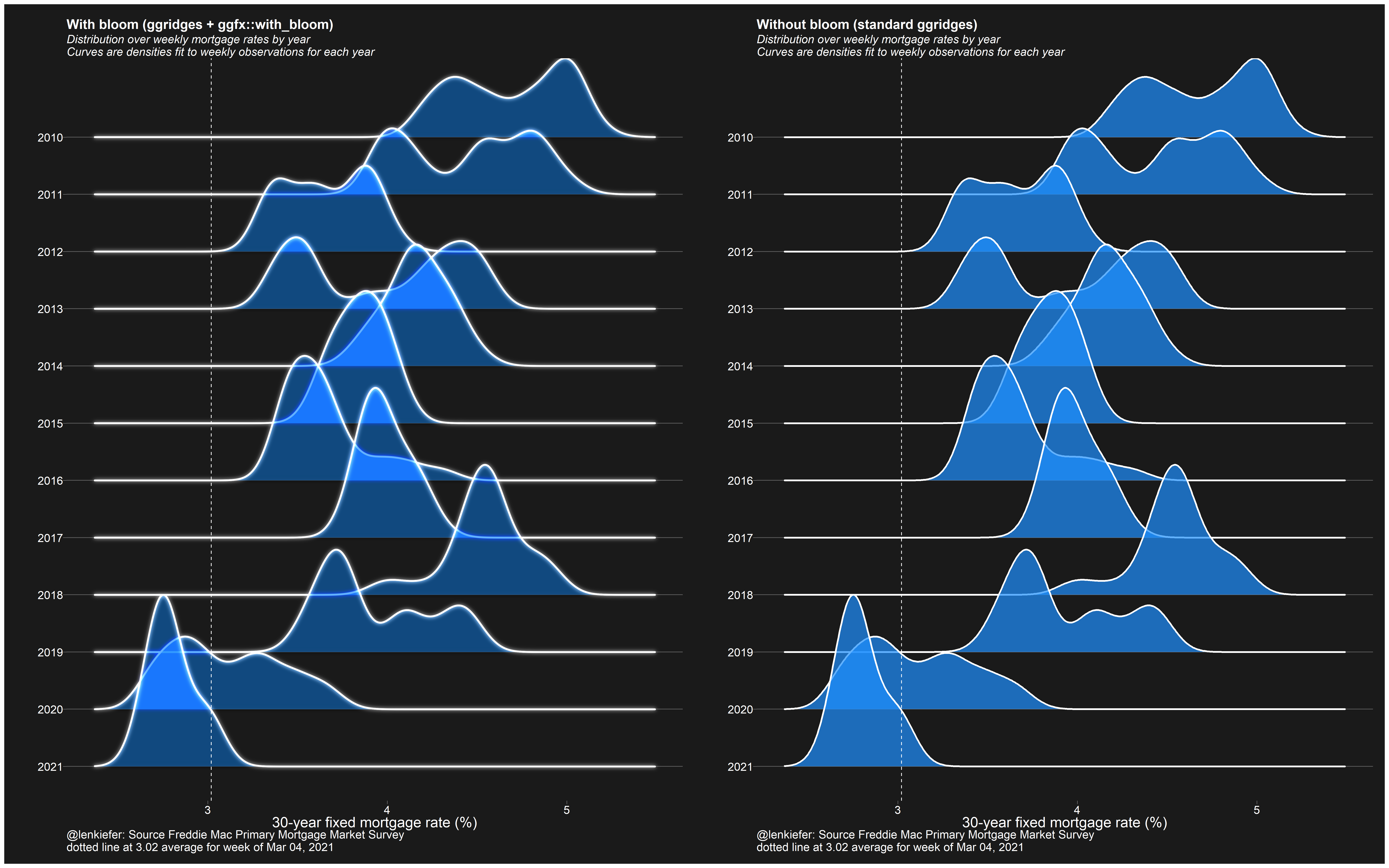# Using ggfx for good

## A possible good use for the new ggfx package

I have been recently messing around with the new ggfx package.

Most of my applications (see below for a gallery) have maybe not been applying good dataviz guidelines. But I think I have found a good example.

We can use the ggfx::with_blend function to layer a recession indicator with a time series and color code the lines. This prevents us from having to use sql like syntax (as we did here) to merge on date ranges.

Instead, we can layer a rectangle with a time series and use the with_blend function to switch the colors depending on whether or not the line falls within a recession region.

First some very brief setup:

library(tidyverse)
library(ggfx)
library(tidyquant)

df <- tidyquant::tq_get("PAYEMS",get="economic.data",from="2000-01-01")
"Peak, Trough
2001-03-01, 2001-11-01
2007-12-01, 2009-06-01
2020-03-02, 2021-04-01"), sep=',',
colClasses=c('Date', 'Date'), header=TRUE)

Then a standard plot with recession shading.

ggplot()+
geom_rect(data=recessions.df,
aes(xmin=Peak,xmax=Trough,ymin=-Inf,ymax=+Inf),
fill='gray',alpha=0.5)+
geom_line(data=df,aes(x=date,y=price),size=0.75,colour ="dodgerblue")+
theme(plot.caption=element_text(hjust=0))+
labs(y="",title="US nonfarm payroll employment (1000s, SA)",
caption=paste0("@lenkiefer Source: U.S. Bureau of Labor Statistics, All Employees,",
"Total Nonfarm [PAYEMS]",
"\nhttps://fred.stlouisfed.org/series/PAYEMS, March 5, 2021."))Now make use of ggfx to blend the recession regions with the line.

ggplot()+
as_reference(geom_rect(data=recessions.df,
aes(xmin=Peak,xmax=Trough,ymin=-Inf,ymax=+Inf),
fill='red'),
id="rec")+
with_blend(
geom_line(data=df,aes(x=date,y=price),size=0.75,colour ="dodgerblue"),
bg_layer = "rec",flip_order=TRUE,
blend_type = 'atop'
)+
theme(plot.caption=element_text(hjust=0))+
labs(y="",title="US nonfarm payroll employment (1000s, SA)",
subtitle="red = recession, blue = expansion",
caption=paste0("@lenkiefer Source: U.S. Bureau of Labor Statistics, All Employees,",
"Total Nonfarm [PAYEMS]",
"\nhttps://fred.stlouisfed.org/series/PAYEMS, March 5, 2021."))## ggfx for not so good

We could also mess around with some other features of ggfx to make some perhaps less good charts.## comparing blur to without blur

Here’s the code for the chart I tweeted out at the start that compares a plot with and without blur.

library(ggridges)
library("ggfx")
library(tidyverse)
library(lubridate)
library(data.table)
library(darklyplot)  # available on github
library(patchwork)

# grab mortgage rate data
df$date <- as.Date(df$date,format="%m/%d/%Y")
df <- mutate(df, yearf=fct_reorder(factor(year(date)), -year(date)))
mycolor <- "#1e90ff"

g1 <-
ggplot(data = filter(df, year(date) > 2009),
aes(y = yearf,
x = pmms30)) +
with_bloom(
geom_density_ridges(
color = "white",
fill = mycolor,
size = 1.1,
scale = 3,
alpha = 0.7
),
colour = "white",
sigma = 10,
keep_alpha = FALSE
) +
darklyplot::theme_dark2(base_family = "Arial", base_size = 18) +
theme(
panel.grid.minor = element_blank(),
plot.title = element_text(size = rel(1.5), face = "bold"),
plot.subtitle = element_text(size = rel(1.3), face = "italic"),
panel.grid.major.x = element_blank()
) +
geom_vline(
data = . %>% tail(1),
linetype = 2,
color = "white",
aes(xintercept = pmms30)
) +
labs(
x = "30-year fixed mortgage rate (%)",
y = "",
title = "With bloom (ggridges + ggfx::with_bloom)",
subtitle = "Distribution over weekly mortgage rates by year\nCurves are densities fit to weekly observations for each year",

caption = paste0(
"@lenkiefer: Source Freddie Mac Primary Mortgage Market Survey\n",
"dotted line at ",
tail(df$pmms30, 1), " average for week of ", format(last(df$date), '%b %d, %Y')
)
)

g2<-
ggplot(data = filter(df, year(date) > 2009),
aes(y = yearf,
x = pmms30)) +
geom_density_ridges(
color = "white",
fill = mycolor,
size = 1.1,
scale = 3,
alpha = 0.7
) +
darklyplot::theme_dark2(base_family = "Arial", base_size = 18) +
theme(
panel.grid.minor = element_blank(),
plot.title = element_text(size = rel(1.5), face = "bold"),
plot.subtitle = element_text(size = rel(1.3), face = "italic"),
panel.grid.major.x = element_blank()
) +
geom_vline(
data = . %>% tail(1),
linetype = 2,
color = "white",
aes(xintercept = pmms30)
) +
labs(
x = "30-year fixed mortgage rate (%)",
y = "",
title = "Without bloom (standard ggridges)",
subtitle = "Distribution over weekly mortgage rates by year\nCurves are densities fit to weekly observations for each year",
caption = paste0(
"@lenkiefer: Source Freddie Mac Primary Mortgage Market Survey\n",
"dotted line at ",
tail(df$pmms30, 1), " average for week of ", format(last(df$date), '%b %d, %Y')
)
)

g1+g2